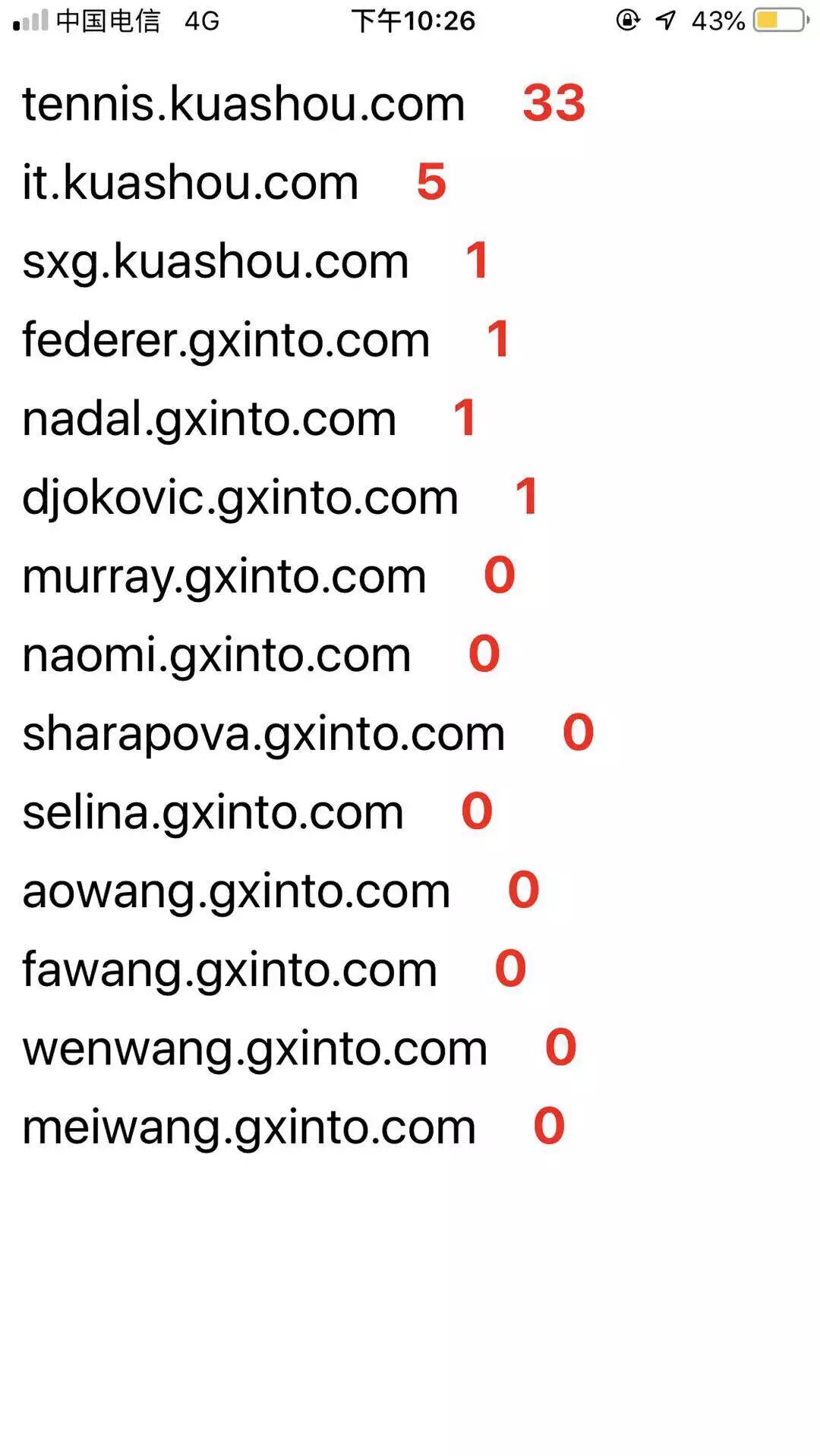百度收录情况查询小应用的实践服务端

• 请求百度的搜索接口
• 处理数据并返回
const axios = require('axios')
const preUrl = 'http://www.baidu.com/s?wd=site:' // 百度搜索接口
let data = await axios.get(`\${preUrl}\${ctx.params.host}`).then(res => res.data)
let reg = /(.*)百度为您找到相关结果约(.*)个(.*)/ //正则匹配返回的html中的搜索结果
let num = data.match(reg)
if(num==null) return ctx.body = 0 //如果匹配不上，说明收录数量为0
ctx.body = num

客户端